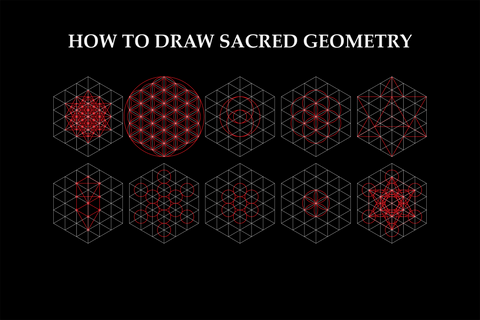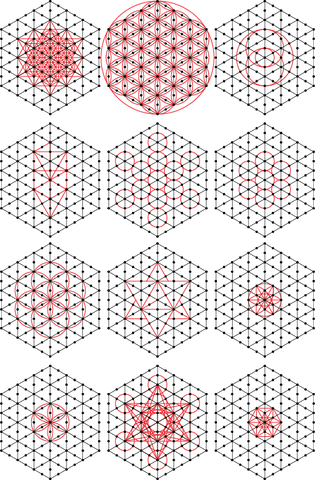# How to Create Sacred Geometry Art: A Step-by-Step GuideIn this guide, you will learn how to create sacred geometry art using a simple step-by-step method. This sacred geometry drawing tutorial will include a downloadable PDF and instructions on drawing various sacred geometry symbols, including the Flower of Life.

Materials you will need:

• Pencil
• Eraser
• Compass
• Straight Edge

## Part 1: How to Draw Sacred Geometry: Understanding the Grid (9 Steps)

The most crucial aspect of this lesson is understanding the fundamental grid from which all primary sacred geometry symbols are drawn. Once you grasp this grid, drawing sacred geometry art becomes surprisingly simple.

1. Draw a vertical line through the middle of your canvas.
2. Using your compass, draw a circle with the origin at the midpoint. Mark a point where the perimeter of the circle overlaps the vertical line.
3. Starting at this point, use your compass to mark four additional equidistant points along the circle's perimeter.
4. Connect all six points to form a hexagon.
5. Divide each side of the hexagon into three segments.
6. Draw a line from the top left and top right corners of the hexagon to the center and divide them into three segments.
7. Erase the lines outside the circle and from the top point of the hexagon to the center.
8. Connect lines from each of the segments to form a Rubik's Cube-like shape.
9. Connect vertical and diagonal lines through each of the points to complete the grid.

## Part 2: How to Draw Sacred Geometry: Understanding How the 12 Symbols Interact with the Grid

In this section, you will learn how each of the Sacred Geometry shapes interacts with the grid you created. Using this grid, you can even freehand draw the shapes with accuracy.

All of the basic sacred geometry shapes fit perfectly into the grid. See examples below:Using these diagrams as reference, you can see how each shape directly corresponds to the points on the grid.